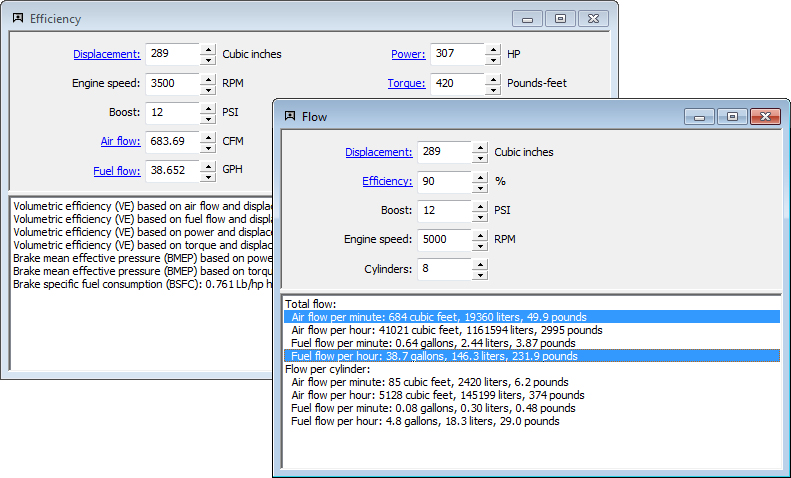Data Transfer

Data transfer in Speed-Wiz works in two different ways. The screen shots below shows two different calculations for the same vehicle to illustrate how it works.The first way that data transfer works is between inputs. Any data that you input in a calculation is automatically updated for all calculations for that vehicle, regardless of whether the other calculations are currently in use (visible on the screen). This is illustrated by the green circles in the screen shot below. Displacement and boost values that were entered in the flow calculation were automatically copied to the inputs for the efficiency calculation. Any calculation that uses any of the five inputs in the flow calculations are automatically updated with the inputs from the flow calculation as they are entered.

The second way that data transfer works is for calculated results. Any calculated result that is used for inputs in other calculations is automatically updated for all calculations for that vehicle, again regardless of whether the other calculations are currently being used. This is illustrated by the red circles in the screen shot below. The calculated air flow and fuel flow from the flow calculation were transferred to the inputs of the efficiency calculation and all other calculations that use flow values for inputs. See Calculation links for more details on calculating input values.